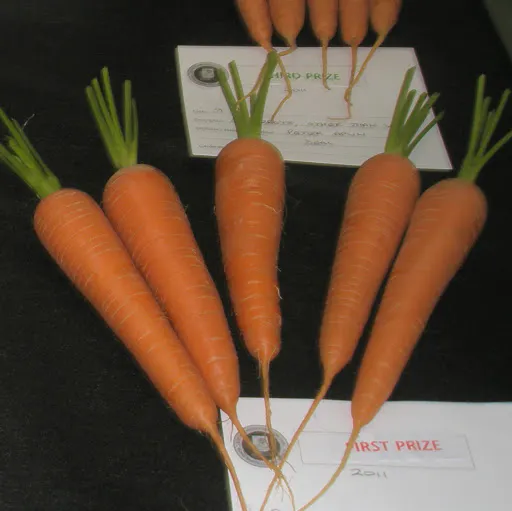Published

# Pheno3D

A low-cost multi-purpose 3D digital photography phenotyping station

IntermediateWork in progress147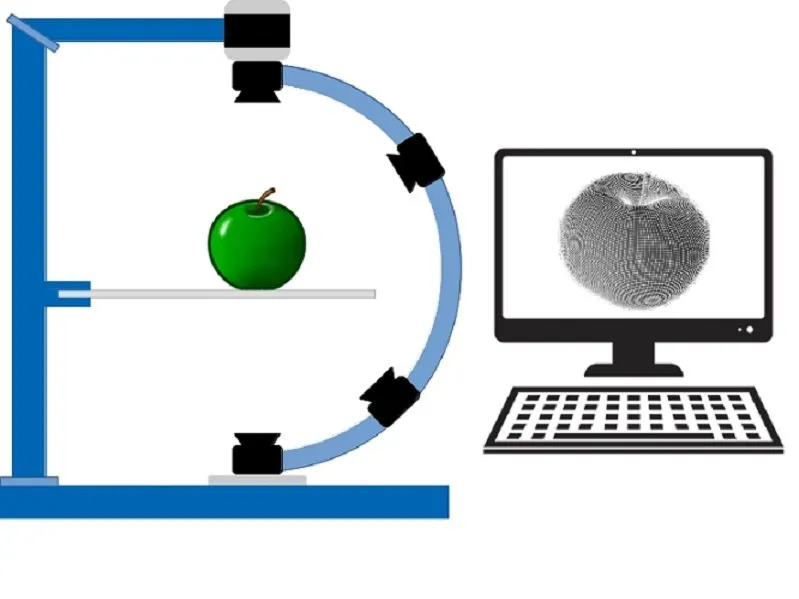## Things used in this project

### Hardware components

 CNBTR 5mm Bore Hole Diameter Brass Worm Gear Shaft + 20 Teeth Worm Wheel 0.5 Modulus Set Drive Gear Box Shaft
×1
 CNBTR 40T Brass Worm Gear Wheel & 5mm Hole Diameter Worm Gear Shaft Kits 0.5 Modulus Set 1:40 Reduction Ratio Drive Gear Box
×1
 Bright Mild Steel Solid Rod Bar 4mm
×1
 M4 (4mm) CAP SCREWS A2 STAINLESS STEEL ALLEN KEY HEXAGON SOCKET BOLTS DIN 912
×1
 Bright Mild Steel Solid Rod Bar 5mm
×1

### Hand tools and fabrication machines3D Printer (generic)

## Schematics

### Frame construction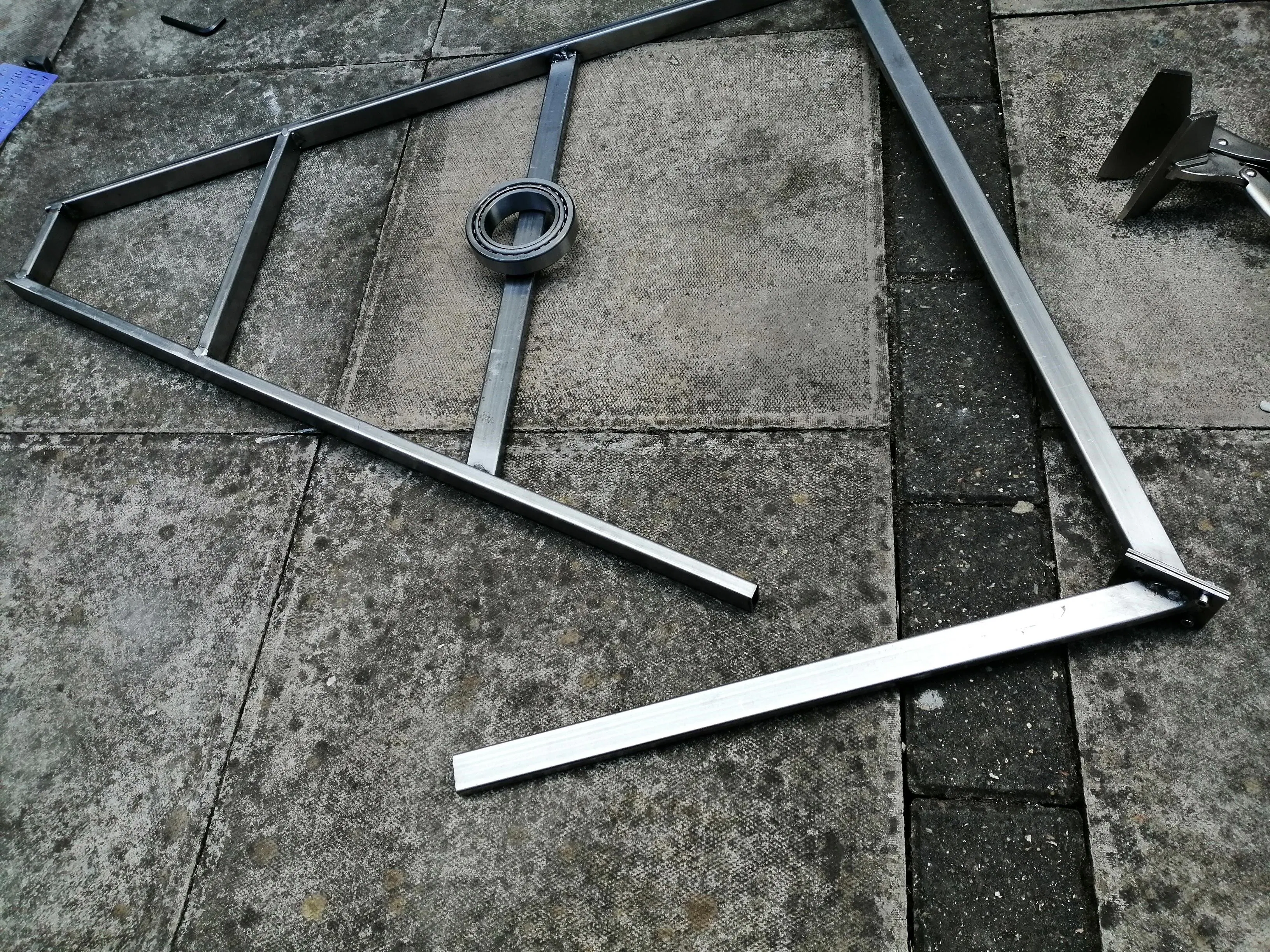### Frame construction### Frame construction

Metal sheets used for welding frame together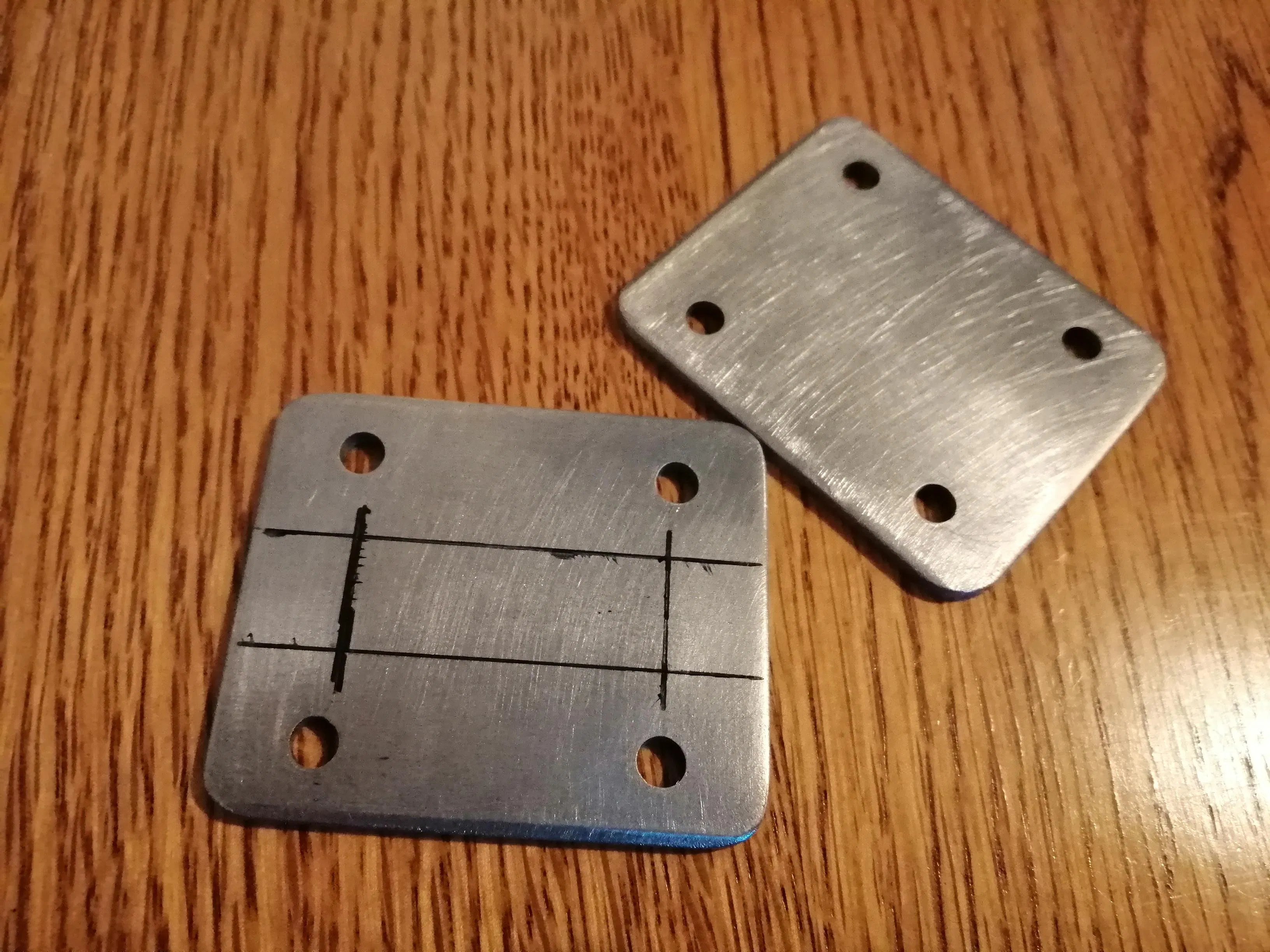### Frame construction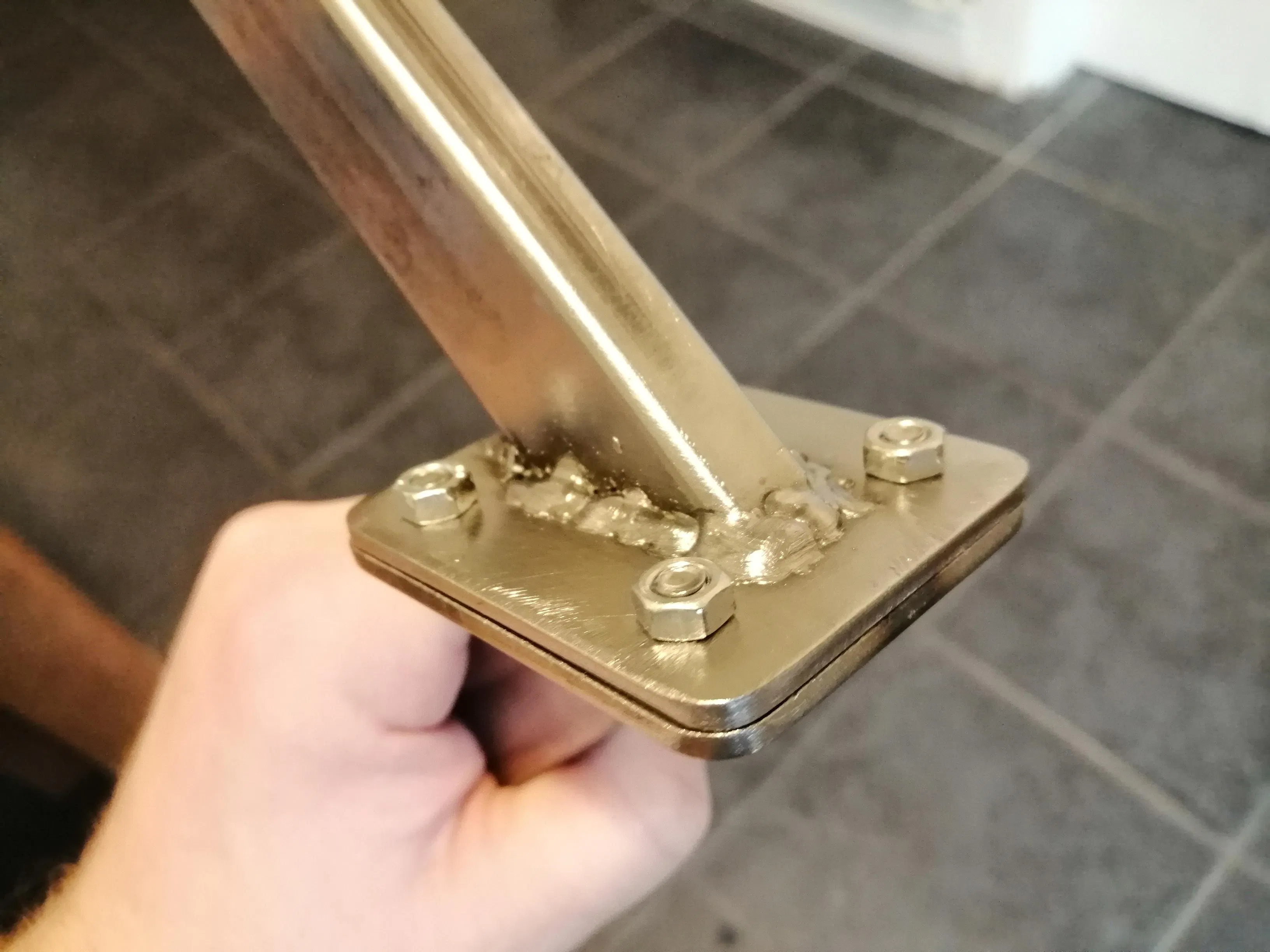### Frame construction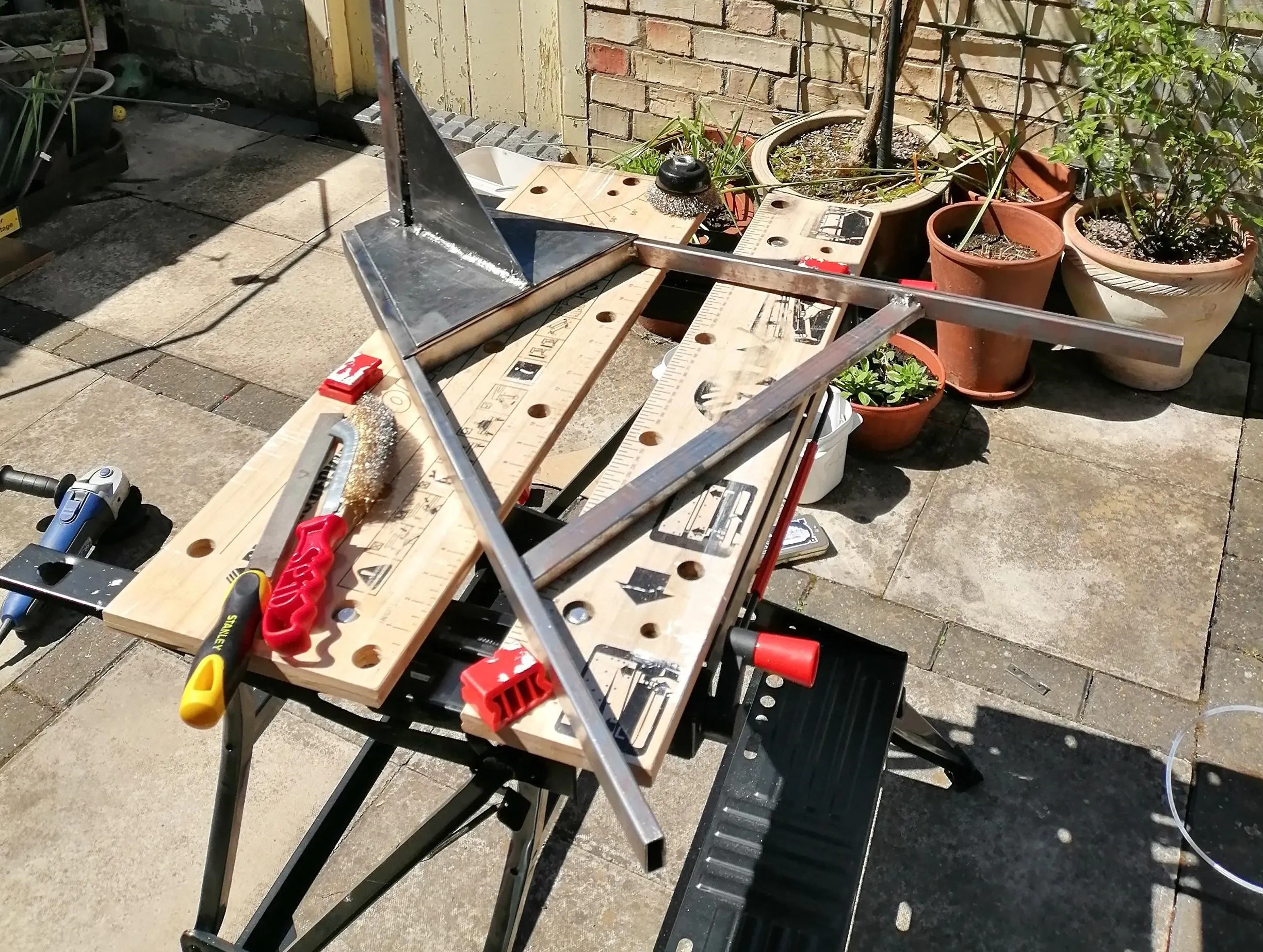## Code

```module motor_housing(){
difference(){
union(){
translate([0,0,0]) cube([47.25,48.5,20]);
translate([1.8,-5.5,0]) cube([3.7,7,20]);
translate([8.63,-3,0]) cube([3,4,20]);
}
union(){
translate([3,3,0]) cube([41.25,42.5,25]);
translate([0,12.25,5]) cube([50,24,20]);
translate([11.625,0,5]) cube([24,50,20]);
}
}
}

module bearing_housing(){
difference(){
union(){
translate([0,0,0]) cylinder(r1 = 15.5, r2 = 13, h = 7, \$fn = 100);
translate([0,0,-2]) cylinder(r = 15.5, h = 2, \$fn = 100);

}
union(){
translate([0,0,0]) cylinder(r = 11.25, h = 7, \$fn = 100);
}
}
}

module base(){
difference(){
union(){
cube([84.2,60,3]);
translate([6,6,3]) cylinder(h = 20, r = 6, \$fn = 100);
//translate([6,54,3]) cylinder(h = 12, r = 6, \$fn = 100);
translate([68.75,54,3]) cylinder(h = 15, r = 6, \$fn = 100);
translate([68.75,6,3]) cylinder(h = 15, r = 6, \$fn = 100);

//translate([68.5,0,0]) cube([0.25,60,5]);
}
union(){
translate([6,6,0]) cylinder(h = 28, r = 2.15, \$fn = 100);
translate([6,54,0]) cylinder(h = 15, r = 2.15, \$fn = 100);
translate([68.75,54,0]) cylinder(h = 18, r = 2.15, \$fn = 100);
translate([68.75,6,0]) cylinder(h = 18, r = 2.15, \$fn = 100);
}
}
}

difference(){
union(){
//cube([100,100,3]);
motor_housing();
//translate([0,24.5,21]) rotate([0,90,0]) cylinder(r = 5, h = 80, \$fn = 100);
translate([60,39,0]) bearing_housing();
//translate([60,39,15]) cylinder(r = 10.5, h = 10, \$fn = 100);
translate([3.75,-12.925,-3]) rotate([0,0,16.5]) base();
}
union(){
translate([60,39,-3]) cylinder(r = 2.6, h= 3, \$fn = 100);
translate([60,39,-0.5]) cylinder(r = 7, h = 1, \$fn = 100);
}
}
```

```module bearing_housing(){
difference(){
union(){
translate([0,0,0]) cylinder(r1 = 15.5, r2 = 13, h = 7, \$fn = 100);
translate([0,0,-3]) cylinder(r = 15.5, h = 3, \$fn = 100);

}
union(){
translate([0,0,0]) cylinder(r = 11.25, h = 7, \$fn = 100);
}
}
}

module base(){
difference(){
union(){
translate([59,0,28])cube([20.2,60,3]);
translate([68.75,54,8]) cylinder(h = 23, r = 6, \$fn = 100);
translate([68.75,6,8]) cylinder(h = 23, r = 6, \$fn = 100);
//translate([68.5,0,0]) cube([0.25,60,5]);
}
union(){
//translate([6,6,0]) cylinder(h = 3, r = 2, \$fn = 100);
//translate([6,54,0]) cylinder(h = 3, r = 2, \$fn = 100);
translate([68.75,54,0]) cylinder(h = 31, r = 2.15, \$fn = 100);
translate([68.75,6,0]) cylinder(h = 31, r = 2.15, \$fn = 100);
}
}
}

difference(){
union(){
//cube([100,100,3]);
//translate([0,24.5,21]) rotate([0,90,0]) cylinder(r = 5, h = 80, \$fn = 100);
translate([60,39,25]) rotate([0,180,0]) bearing_housing();
//translate([60,39,15]) cylinder(r = 10.5, h = 10, \$fn = 100);
translate([3.75,-12.925,-3]) rotate([0,0,16.5]) base();

//translate([60,39,25]) cylinder(r = 2.7, h= 3, \$fn = 100);
//translate([60,39,-1]) cylinder(r = 7, h = 1, \$fn = 100);
}
union(){
translate([60,39,25]) cylinder(r = 2.6, h= 3, \$fn = 100);
translate([60,39,25]) cylinder(r = 7, h = 0.5, \$fn = 100);
}
}
```

```difference(){
union(){
cylinder(r = 6, h = 7, \$fn = 150); // was 7.5 changed 23rd June not printed
translate([79,0,0]) cylinder(r = 6, h = 25.5, \$fn = 150);
translate([0,-6,0]) cube([78.5,12,3]);
}
union(){
cylinder(r = 2.15, h = 7, \$fn = 150);
translate([79,0,0]) cylinder(r = 2.15, h = 25.5, \$fn = 150);
}
}
```

### Worm gear spacer OpenSCAD code

```difference(){
union(){
cylinder(r = 7.5, h= 4, \$fn = 160);
translate([0,0,4]) cylinder(r = 6, h= 0.25, \$fn = 160);
translate([0,0,4.25]) cylinder(r = 4, h= 6.75, \$fn = 160);
}
union(){
cylinder(r = 2.7, h= 12, \$fn = 160);
}
}
```

## Credits

### Yeorgia Argirou

2 projects • 0 followers

### Lawrence Percival-Alwyn

5 projects • 3 followers

### Richard Harrison

2 projects • 0 followers

### Sam Mugford

4 projects • 2 followers

### Graham Taylor

0 projects • 0 followers